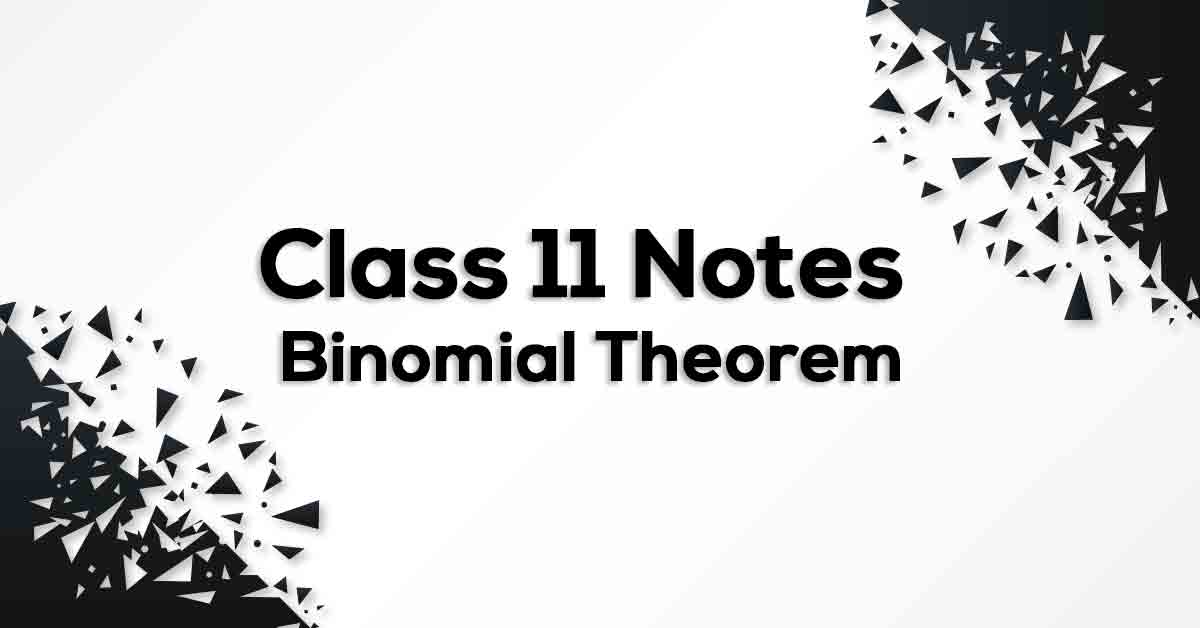Binomial Theorem Class 11 Formulas & Notes | Vidyakul
×

# Binomial Theorem Class 11 Formulas & Notes## Class 11 Math Chapter 8 Binomial Theorem Formulas - PDF Download

Binomial Theorem Class 11 Formulae & Notes is prepared strictly according to the NCERT Syllabus which not only reduces the pressure on the students but also, offer them a simple way to study or revise the chapter. These formulae are cumulated from past 15 years of examination material preferred by CBSE so that no important formulae should be left behind for the students to know and practice. Binomial Theorem Class 11 Formulas & Notes would fuel your exam preparation and increase your self-confidence which would help you score maximum marks in the exam.

### What do you understand by Binomial Theorem?

We know how to find the squares and cubes of binomials like a + b and a – b. E.g. (a+b)2, (a-b)3  etc. However, for higher powers calculation becomes difficult. This difficulty was overcome by a theorem known as binomial theorem. It gives an easier way to expand (a + b)n, where n is an integer or a rational number. Total number of terms in expansion = index count +1.  g. expansion of (a + b)2, has 3 terms. Powers of the first quantity ‘a’ go on decreasing by 1 whereas the powers of the second quantity ‘b’ increase by 1, in the successive terms. In each term of the expansion, the sum of the indices of a and b is the same and is equal to the index of a + b.

Know more about the same in Binomial Theorem Class 11 Formulas & Notes pdf.